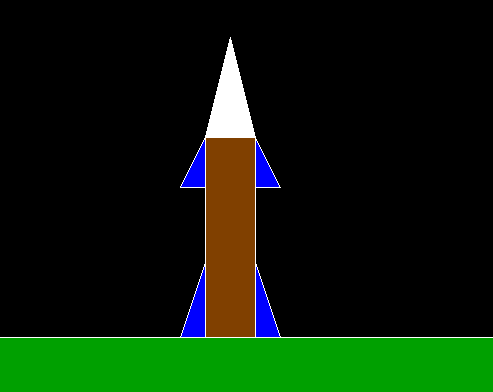Skip to content
Related Articles
C program to create a Rocket using Graphics
• Last Updated : 01 Apr, 2021

In this article, we will discuss how to draw the Rocket using Graphics.

Approach:

• Draw a straight line using the line() function that will act as the Ground Outline.
• Below the above line drawn, fill it with the color green using two functions setfillstyle() and floodfill().
• Create a rectangle using the rectangle() function and color it brown that will act as the rocket Body.
• Create the total four triangles by using the line() function which will act as the wing of the rocket to make it appear more attractive.
• Among them, two will be situated at the two sides of the upper part of the rocket & the lower part of the rocket.
• Color all the wings with blue color.
• Create a triangle using the line() that will act as the head of the rocket. and color it white.

Below is the implementation of the above approach:

## C

 `// C program for the above approach ` ` `  `#include ` `#include ` `#include ` ` `  `// Driver Code ` `void` `main() ` `{ ` `    ``// Initialize of gdriver with ` `    ``// DETECT macros ` `    ``initgraph(&gd, &gm, ``"C:\\turboc3\\bgi"``); ` ` `  `    ``setfillstyle(SOLID_FILL, BROWN); ` `    ``rectangle(650, 450, 700, 650); ` ` `  `    ``// Rocket body ` `    ``floodfill(652, 648, 15); ` `    ``line(0, 650, 1500, 650); ` ` `  `    ``// Land outline ` `    ``setfillstyle(SOLID_FILL, WHITE); ` `    ``line(650, 450, 700, 450); ` `    ``line(650, 450, 675, 350); ` `    ``line(675, 350, 700, 450); ` ` `  `    ``// Rocket head ` `    ``floodfill(652, 448, 15); ` `    ``setfillstyle(SOLID_FILL, BLUE); ` `    ``line(650, 500, 650, 450); ` `    ``line(625, 500, 650, 500); ` `    ``line(625, 500, 650, 450); ` ` `  `    ``// Rocket left up wing ` `    ``floodfill(648, 498, 15); ` `    ``setfillstyle(SOLID_FILL, BLUE); ` `    ``line(700, 450, 700, 500); ` `    ``line(725, 500, 700, 500); ` `    ``line(700, 450, 725, 500); ` ` `  `    ``// Rocket right up wing ` `    ``floodfill(702, 498, 15); ` `    ``setfillstyle(SOLID_FILL, BLUE); ` `    ``line(625, 650, 650, 650); ` `    ``line(650, 575, 650, 650); ` `    ``line(625, 650, 650, 575); ` ` `  `    ``// Rocket left down wing ` `    ``floodfill(627, 648, 15); ` `    ``setfillstyle(SOLID_FILL, GREEN); ` `    ``floodfill(627, 698, 15); ` ` `  `    ``setfillstyle(SOLID_FILL, BLUE); ` `    ``line(725, 650, 700, 650); ` `    ``line(700, 575, 700, 650); ` `    ``line(725, 650, 700, 575); ` ` `  `    ``// Rocket right down wing ` `    ``floodfill(702, 648, 15); ` `    ``line(0, 650, 1500, 650); ` ` `  `    ``// Draw the land with green color ` `    ``setfillstyle(SOLID_FILL, GREEN); ` `    ``floodfill(627, 698, 15); ` `    ``getch(); ` ` `  `    ``// Close the initialized gdriver ` `    ``closegraph(); ` `} `

Output:Want to learn from the best curated videos and practice problems, check out the C Foundation Course for Basic to Advanced C.

My Personal Notes arrow_drop_up
Recommended Articles
Page :